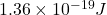## The threshold wavelength for the photoelectric effect for silver is 262 nm. (a) Determine the work function for silver. (b) What is the maxi

Question

The threshold wavelength for the photoelectric effect for silver is 262 nm. (a) Determine the work function for silver. (b) What is the maximum kinetic energy of an electron emitted from silver if the incident light has a wavelength of 222 nm

in progress 0
2 months 2021-07-19T07:50:36+00:00 1 Answers 0 views 0

We are given that

Threshold wavelength,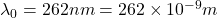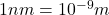a.Work function,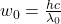Where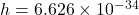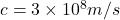Using the formula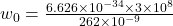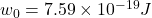b.Wavelength,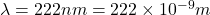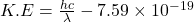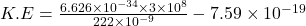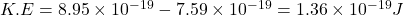Hence, the maximum kinetic energy of an electron emitted=